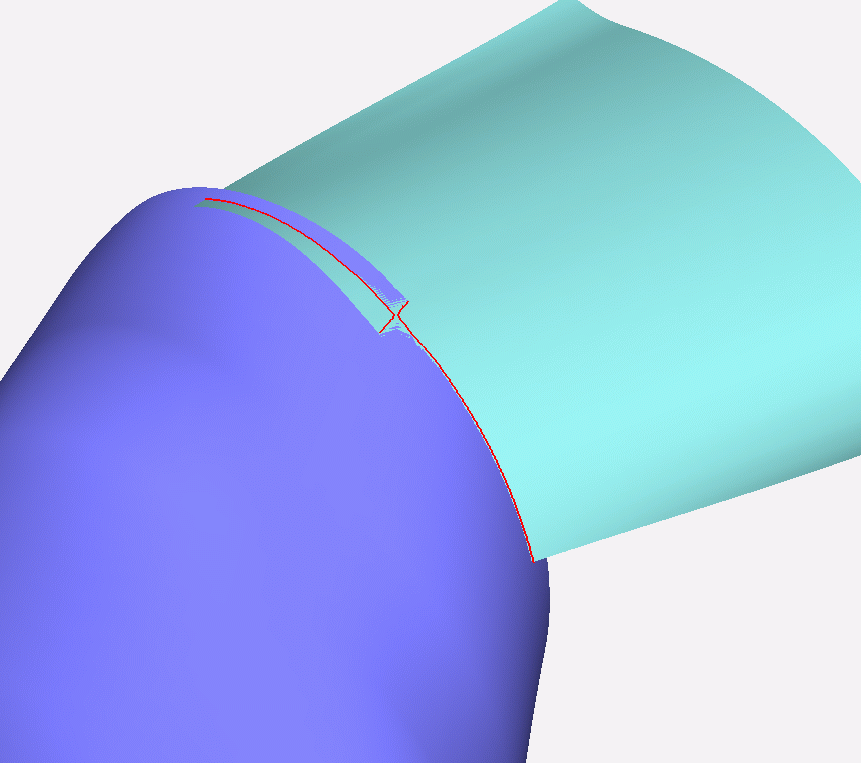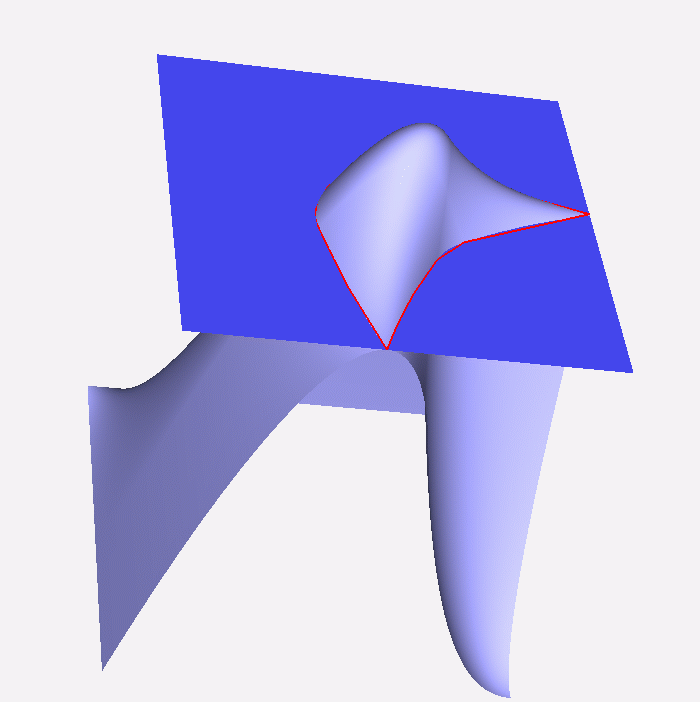GAIA Home Intersections in CAD Change of representation Intersection algorithms in GAIA Algebraic geometry for CAD Applications Software The GAIA project Intersection challenges When surfaces intersected are parallel or near parallel close to the intersection, implementing intersection algorithms becomes a challenge. Simple techniques such as triangulating the surfaces before intersecting or using lattice evaluation have no guarantee of working. In these situations, traditional recursive subdivision based intersection algorithms will often run for a long time before either concluding that no result can be found or returning a result. Intersections where the surfaces are parallel close to the intersection curve arise in many situations:Two surfaces sharing a common edge are intersected. If the edge represents a trimming curve in both surfaces and the transition over the edge is smooth, then we have a near singular situation. In CAD-systems the information in the boundary structure can be used to avoid this situation. But when receiving a CAD-model on a neutral format such information is often lost.A blending surface is intersected with a parent surface used when defining the blend.A sculptured surface is intersected with its offset surface.Intersections of lofting surfaces that interpolate a number of common curves, but in addition, each lofting interpolates some other curves.In general, designed objects often have smooth transitions between surface regions. So when designing the object by CAD, the surfaces composing the outer and the inner shells have to be represented in a CAD-model with many smooth transitions between adjacent faces (surface elements). The example to the left shows two parallel surfaces that do not intersect. For such surfaces, traditional recursive algorithms will use a lot of time before deciding that no intersection exists. A triangulation base method might produce false intersections. A lattice evaluation method will be fairly fast and decide that no intersection can be found. However, lattice evaluation might always miss out intersections.   Published June 7, 2005The correct intersection is the red curve. The triangulation supplied for the visualization shows a different "visual" intersection topology. An example showing that a triangulation based approach will provide incorrect results in near singular intersections.Most of the intersection curve is transversal. However, there are two singular points on the intersection curve.